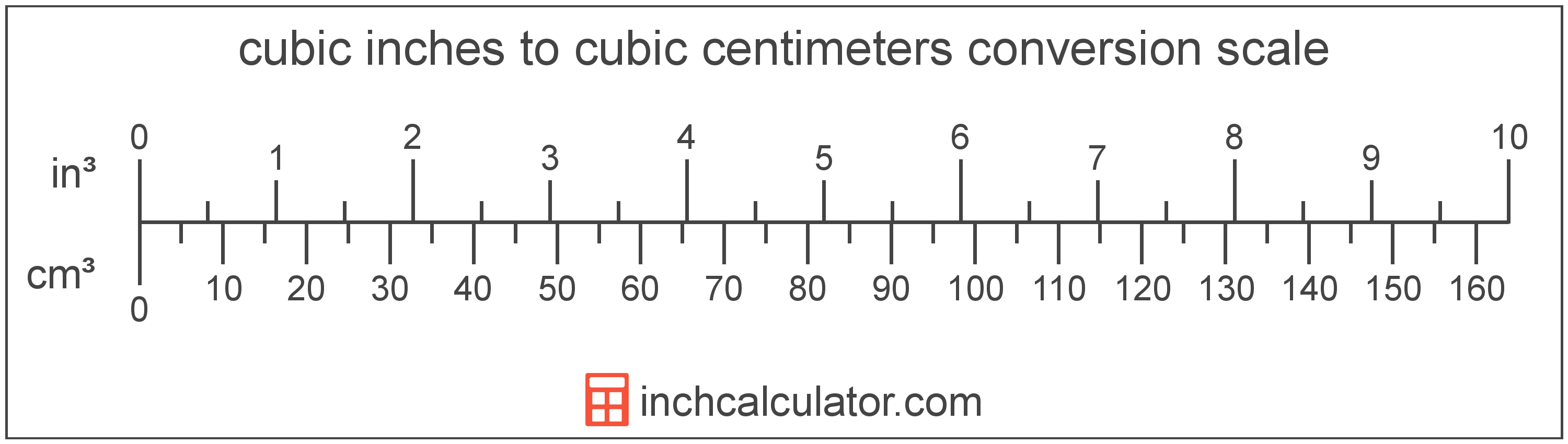# Cubic Centimeters to Cubic Inches Conversion

Enter the volume in cubic centimeters below to get the value converted to cubic inches.

Results in Cubic Inches:1 cm³ = 0.061024 in³

## How to Convert Cubic Centimeters to Cubic InchesTo convert a cubic centimeter measurement to a cubic inch measurement, multiply the volume by the conversion ratio.

Since one cubic centimeter is equal to 0.061024 cubic inches, you can use this simple formula to convert:

cubic inches = cubic centimeters × 0.061024

The volume in cubic inches is equal to the cubic centimeters multiplied by 0.061024.

For example, here's how to convert 5 cubic centimeters to cubic inches using the formula above.
5 cm³ = (5 × 0.061024) = 0.305119 in³

## Cubic Centimeters

The cubic centimeter is a unit that is equal to the volume of a cube with one centimeter sides. One cubic centimeter is equal to about 0.061 cubic inches.

The cubic centimeter, or cubic centimetre, is a multiple of the cubic meter, which is the SI derived unit for volume. In the metric system, "centi" is the prefix for 10-2. Cubic centimeters can be abbreviated as cm³, and are also sometimes abbreviated as cu cm, cc, or ccm. For example, 1 cubic centimeter can be written as 1 cm³, 1 cu cm, 1 cc, or 1 ccm.

## Cubic Inches

A cubic inch is a unit of volume equal to the space consumed by a cube with sides that are one inch in all directions. One cubic inch is equivalent to about 16.387 cubic centimeters or 0.554 fluid ounces.

The cubic inch is a US customary and imperial unit of volume. A cubic inch is sometimes also referred to as a cubic in. Cubic inches can be abbreviated as in³, and are also sometimes abbreviated as cu inch, cu in, or CI. For example, 1 cubic inch can be written as 1 in³, 1 cu inch, 1 cu in, or 1 CI.

## Cubic Centimeter to Cubic Inch Conversion Table

Cubic centimeter measurements converted to cubic inches
Cubic Centimeters Cubic Inches
1 cm³ 0.061024 in³
2 cm³ 0.122047 in³
3 cm³ 0.183071 in³
4 cm³ 0.244095 in³
5 cm³ 0.305119 in³
6 cm³ 0.366142 in³
7 cm³ 0.427166 in³
8 cm³ 0.48819 in³
9 cm³ 0.549214 in³
10 cm³ 0.610237 in³
11 cm³ 0.671261 in³
12 cm³ 0.732285 in³
13 cm³ 0.793309 in³
14 cm³ 0.854332 in³
15 cm³ 0.915356 in³
16 cm³ 0.97638 in³
17 cm³ 1.0374 in³
18 cm³ 1.0984 in³
19 cm³ 1.1595 in³
20 cm³ 1.2205 in³
21 cm³ 1.2815 in³
22 cm³ 1.3425 in³
23 cm³ 1.4035 in³
24 cm³ 1.4646 in³
25 cm³ 1.5256 in³
26 cm³ 1.5866 in³
27 cm³ 1.6476 in³
28 cm³ 1.7087 in³
29 cm³ 1.7697 in³
30 cm³ 1.8307 in³
31 cm³ 1.8917 in³
32 cm³ 1.9528 in³
33 cm³ 2.0138 in³
34 cm³ 2.0748 in³
35 cm³ 2.1358 in³
36 cm³ 2.1969 in³
37 cm³ 2.2579 in³
38 cm³ 2.3189 in³
39 cm³ 2.3799 in³
40 cm³ 2.441 in³

## References

1. National Institute of Standards and Technology, Specifications, Tolerances, and Other Technical Requirements for Weighing and Measuring Devices, Handbook 44 - 2019 Edition, https://nvlpubs.nist.gov/nistpubs/hb/2019/NIST.HB.44-2019.pdf
2. National Institute of Standards and Technology, Specifications, Tolerances, and Other Technical Requirements for Weighing and Measuring Devices, Handbook 44 - 2019 Edition, https://nvlpubs.nist.gov/nistpubs/hb/2019/NIST.HB.44-2019.pdf